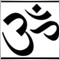# Need help with OrderClose() and order limiting21
auto_free_listep

Hi all,

I'm new to MQL4 and have been working on my very first EA, which is Ichimoku based. The robot seems to open the trade at the proper signal, but then keeps opening trades all the way down the bar. The trades are not closed until the very end of the testing period.

I have tried setting a boolean operator (OpenOrder) to make the EA trade only when OpenOrder==False, but for the life of me cannot figure out what is going wrong and where. I have a feeling I'm missing something obvious.

As for closing the order, I'm not exactly sure what's going wrong.

If anyone could nudge me a little closer to parsimony I would be eternally grateful.

```//+------------------------------------------------------------------+
//|                                              Ichimoku Custom.mq4 |
//|                                                           Luke   |
//|                                                                  |
//+------------------------------------------------------------------+
#include <stdlib.mqh>
#include <stderror.mqh>
#define SIGNAL_NONE 0
#define SIGNAL_SELL  2
#define SIGNAL_CLOSESELL 4

//---- input parameters
extern int       StopLoss=0;
extern int       TakeProfit=0;
extern double    Lots=0.1;
extern double    UserTrailingStop;
//------ Initialization function
int init()
{
return(0);
}
//------ Deinitialization function
int deinit()
{
return(0);
}
//+------------------------------------------------------------------+
//| expert start function                                            |
//+------------------------------------------------------------------+
int start()
{
double tenkanSen = iIchimoku(NULL, 0, 9, 26, 52, MODE_TENKANSEN, 0);
double kijunSen = iIchimoku(NULL, 0, 9, 26, 52, MODE_KIJUNSEN, 0);
double senkouA = iIchimoku(NULL, 0, 9, 26, 52, MODE_SENKOUSPANA, 26);
double senkouB = iIchimoku(NULL, 0, 9, 26, 52, MODE_SENKOUSPANB, 26);
double openSen = iOpen(NULL, 0, 0);
double chinkou = iIchimoku(NULL, 0, 9, 26, 52, MODE_CHINKOUSPAN, 0);
double closeSen = iClose(NULL, 0, 0);
double highSen = iHigh(NULL, 0, 26);
double RSIsen = iRSI(NULL, 0, 14, PRICE_CLOSE, 0);
string symbol;
bool OpenOrder = False;
int order = SIGNAL_NONE;
int ticket, total, cnt, type;
double SL,TP;
//--------------------------------------------------------------------------------//
//                                                                                //
//                           Define Buy & Sell                                    //
//                                                                                //
//--------------------------------------------------------------------------------//
if (tenkanSen > kijunSen && openSen > senkouA && openSen > senkouB && chinkou > highSen && RSIsen > 50) order = SIGNAL_BUY; // Buy Parameters
if (order==1 && OpenOrder==False) // If there is a BUY signal
{
if (StopLoss!=0)    SL=Bid-StopLoss*Point;   else SL=0;  // define SL
if (TakeProfit!=0)  TP=Bid+TakeProfit*Point; else TP=0;  // Define TP
if(ticket>0)
{
OpenOrder=True;
symbol = OrderSymbol();
}
else
{
Print("Error opening BUY order : ", GetLastError());
}
}
if (tenkanSen < kijunSen && openSen < senkouA && openSen < senkouB && chinkou < highSen && RSIsen < 50) order = SIGNAL_SELL; // Sell Parameters
if (order==2 && OpenOrder==False) // ??????? ???????, ??????? ???????
{
ticket = OrderSend(Symbol(),OP_SELL,Lots,Bid,3,SL,TP);
type = OP_SELL;
if(ticket>0)
{
if(OrderSelect(ticket,SELECT_BY_TICKET,MODE_TRADES)) Print("SELL order opened : ",OrderOpenPrice());
OpenOrder = True;
symbol = OrderSymbol();
}
else
{
Print("Error opening SELL order : ", GetLastError());
}
}
// if(tenkanSen < kijunSen || openSen < kijunSen) order = SIGNAL_CLOSEBUY;
// if(tenkanSen > kijunSen || openSen > kijunSen) order = SIGNAL_CLOSESELL;
//--------------------------------------------------------------------------------//
//                                                                                //
//                           Close Position                                       //
//                                                                                //
//--------------------------------------------------------------------------------//

if(OpenOrder==True)
{
if((OrderType()==0 || OrderType()==1) &&   // check for opened position
OrderSymbol()==symbol)  // check for symbol
{
if(type==0) // if long position is open
{
if(tenkanSen < kijunSen || openSen < kijunSen) order = SIGNAL_CLOSEBUY; // Should BUY be closed?
if(order == 3)
{
if(OrderClose(ticket,OrderLots(),Bid,3,Red)==True) ticket = 0; // close position
OpenOrder=False;
if(ticket==0) // verify the position was closed
{
total--;
}
return(0); // exit
}
}
if(type==1) // if short position is open
{
if(tenkanSen > kijunSen || openSen > kijunSen) order = SIGNAL_CLOSESELL; // should SELL be closed?
if(order == 4)
{
if(OrderClose(ticket,OrderLots(),Bid,3,Red)==True) ticket = 0; // close position
OpenOrder = False;
if(ticket==0)
{
if(OrderSelect(ticket,SELECT_BY_TICKET,MODE_TRADES)==True) Print("SELL closed at: ", OrderClosePrice());
total--;
}
return(0); // exit
}
}
if(type!=1 && type!=0)
{
Print("Error closing order : ", GetLastError());
}
}

} // closes loop

//----

return(0);
}
//+------------------------------------------------------------------+
```5299

Your "OpenOrder" needs to be a static variable. Static variables  and Global [ scope ] variables saves their value between ticks. local variables do-not. The problem is you'll have to remember to re-set these variables back to false when there's no-longer an order. Most people would just count the number of open-orders.

Some example below. The function version would be considered the most reliable.

```/*Example using Static Variables.*/
void start(){
static bool OpenOrder;
//static variable Inside the start function
if(OpenOrder==false){
if(OrderSend(...)>-1){OpenOrder=true;}
}

if(OpenOrder==true){
if(OrderClose(...)){OpenOrder=false;}
}
}

/*Example using Global [scope] Variables.*/
bool OpenOrder;
//Global variable Outside the start function
void start(){
if(OpenOrder==false){
if(OrderSend(...)>-1){OpenOrder=true;}
}
if(OpenOrder==true){
if(OrderClose(...)==true){OpenOrder=false;}
}
}

/*Example using function to count your orders.*/
void start(){
if(MyOrderCount()==0){OrderSend(...);}
if(MyOrderCount() >0){OrderClose(...);}
}```21
auto_free_listep
ubzen:

Your "OpenOrder" needs to be a static variable. Static variables  and Global [ scope ] variables saves their value between ticks. local variables do-not. The problem is you'll have to remember to re-set these variables back to false then there's no-longer an order. Most people would just count the number of open-orders.

Some example below. The function version would be considered the most reliable.

Thank you! That saved me. I will try coding the order counting function in and posting it here.